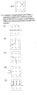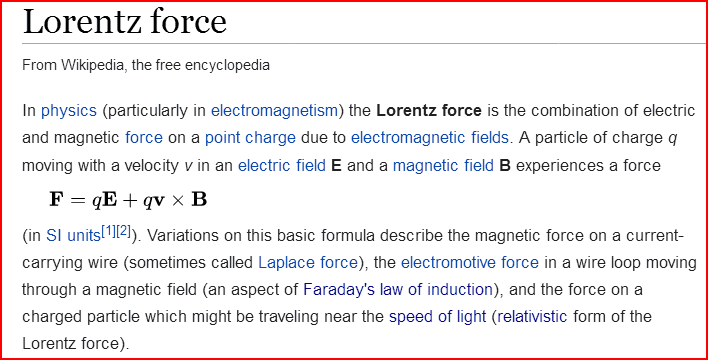# Magnetic force direction on a positive and negative charges

• YMMMA
In summary, the question asks about the path of the electron. The Attempt at a Solution states that if it is an electron, the left hand rule should be followed.f

## Homework Statement

The question askes about the path of the electron. I need to know if I am doing it correctly whether it was asked for a proton or an electron.

F=QV⊥Bsinθ

## The Attempt at a Solution

I answered it C. Because it is an electron, I used my left hand. My thumb points to the right and my other fingers pointing into the page, so the force points to the bottom of the page. Thus, the electron’s will be as illustrated in choice C. If that was a proton, it will be the other way around, like in B. Am I right?

#### Attachments

•D164161B-D704-4A1E-84BB-C72DA7FDC5B2.jpeg
13.9 KB · Views: 296
The answer C is correct, but I always use the "Right Hand Rule" and am careful about the sign of the charge. If it is negative, then the Qv vector term points in the opposite direction from the velocity vector. Does that make sense? Are you familiar with the vector version of the Lorentz Force?

https://en.wikipedia.org/wiki/Lorentz_force#### Attachments

The answer C is correct, but I always use the "Right Hand Rule" and am careful about the sign of the charge. If it is negative, then the Qv vector term points in the opposite direction from the velocity vector. Does that make sense? Are you familiar with the vector version of the Lorentz Force?

https://en.wikipedia.org/wiki/Lorentz_force

View attachment 232262
I am, yes. But does that means reversing the direction of Qv vector is the same if I used my left hand.
I take it as a rule: left hand if it’s an electron, right hand if it’s a proton.

that seems to be correct, yes. you can still use your right hand, though, just point the thumb in the opposite direction of the motion of the negative charge and the middle finger will show the direction of the force. but when you are confronted with a drawing requiring you to twist your hand in an "impossible" way it is easier to rather turn the drawing to make life easier•YMMMA and berkeman
I am, yes. But does that means reversing the direction of Qv vector is the same if I used my left hand.
I take it as a rule: left hand if it’s an electron, right hand if it’s a proton.
I guess you can do that, but it's easier for me to just always use my right hand and be careful about the sign of the charge. Whichever works for you I guess.

•YMMMA
Got it. Thank you all.

•berkeman